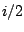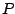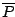Next: Fourier analysis and resynthesis Up: Filters Previous: Making and using all-pass   Contents   Index

# Exercises

1. A recirculating elementary filter has a pole at. At what angular frequency is its gain greatest, and what is the gain there? At what angular frequency is the gain least, and what is the gain there?

2. A shelving filter has a pole at 0.9 and a zero at 0.8. What are: the DC gain; the gain at Nyquist; the approximate transition frequency?

3. Suppose a complex recirculating filter has a pole at. Suppose further that you want to combine its real and imaginary output to make a single, real-valued signal equivalent to a two-pole filter with poles atand. How would you weight the two outputs?

4. Suppose you wish to design a peaking filter with gain 2 at 1000 Hertz and bandwidth 200 Hertz (at a sample rate of 44100 Hertz). Where, approximately, would you put the upper pole and zero?

5. In the same situation, where would you put the (upper) pole and zero to remove a sinusoid at 1000 Hertz entirely, while attenuating only 3 decibels at 1001 Hertz?

6. A one-pole complex filter is excited by an impulse to make a tone at 1000 Hertz, which decays 10 decibels in one second (at a sample rate of 44100 Hertz). Where would you place the pole? What is the value of q"?Next: Fourier analysis and resynthesis Up: Filters Previous: Making and using all-pass   Contents   Index
Miller Puckette 2006-12-30